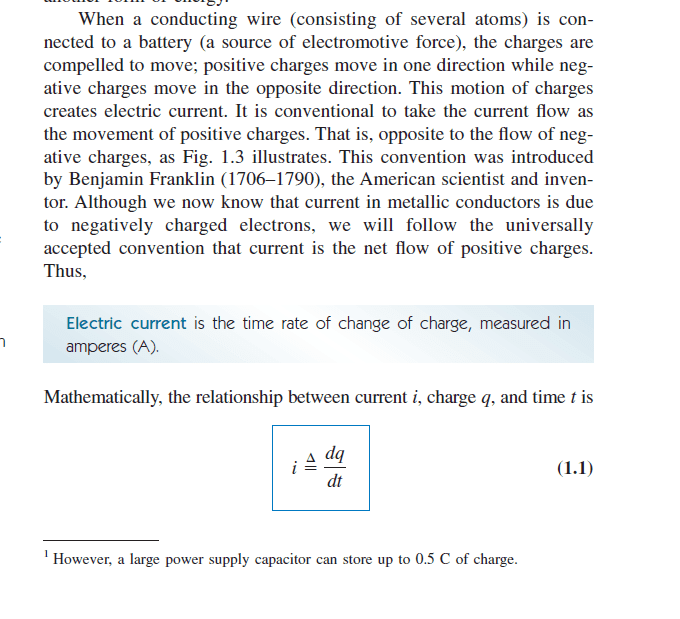# Why is current defined as the rate of change of charge?

• CoolDude420

#### CoolDude420

Homework Statement:: This isn't a homework question but just a theoretical questions.

[mentor’s note: moved to a more appropriate forum for theoretical questions.]

I know that current is defined as the rate of change of charge per unit time.
i = dq/dt

This makes sense for a capacitor which stores charge. If we define the q as being the total charge stored on the capacitor, then sure, the rate of change of the total q stored on the capacitor will tell you how much current the capacitor is receiving.

However, in this case of a resistor, this confuses me. A resistor does not store charge. If I have a DC voltage source connected across a resistor, the resistor technically should be receiving the same amount of charge for all time, hence the rate of change of charge dq/dt will always be 0, thus, i = dq/dt = 0. Does this mean that the resistor is receiving 0 current?

Where is the flaw in my understanding? I think I might be confusing instantaneous charge flowing versus total charge?
Relevant Equations:: i = dq/dt

V = IR

N/A

Last edited by a moderator:
Where is the flaw in my understanding? I think I might be confusing instantaneous charge flowing versus total charge?
You are. If you use a hose to fill a bucket with water, the bucket at any time will contain ##m## kilograms of water and the hose is filling it at the rate of ##\frac{dm}{dt}## kilograms per minute. If you are charging a capacitor (bucket) using a conducting wire (hose), the capacitor at any time will have ##Q## Coulombs of charge and the wire is charging it at the rate of ##\frac{dQ}{dt}## Coulombs per minute.

Please post in the regular forums when you have questions that are not homework or homework-like problems. Also, putting all of your text in the homework statement section makes it impossible to quote selected parts of it in a simple manner.

Where did you read that current is defined this way? It is not true. It is true, as you mentioned, for a capacitor (but then only for the charge on one of the plates - the total charge remains zero), but more generally current is the flow of charge per unit time.

Please post in the regular forums when you have questions that are not homework or homework-like problems. Also, putting all of your text in the homework statement section makes it impossible to quote selected parts of it in a simple manner.

Where did you read that current is defined this way? It is not true. It is true, as you mentioned, for a capacitor (but then only for the charge on one of the plates - the total charge remains zero), but more generally current is the flow of charge per unit time.

Sorry, I apologies for not posting correctly.Fundamentals of Electric Circuits Book

It may be easier for you to think of current defined as charges crossing a surface. So current is the number of charges crossing per time unit. Like charge flux.

You can define some surface in the middle of that resistor and measure the rate of charges moving across that surface. Same definition with wires, inductors, capacitors, and the solar wind in space. Yes, the capacitor stores those charges, but it's the flow (flux) you are measuring, not what happens to them, or where they end up.

PS: Sorry, I was sloppy about flow vs. flux. Flow (current) is charges/sec across a defined area. Flux (current density) is charges/(sec⋅area) at any part of the surface. Similar concepts though.

Last edited:
•vanhees71
The charges are either going in or out of the capacitor through the only way in (or out). But with a resistance, there is one way in and one way out. Whatever goes in must come out such that ##i_{in} = i_{out}## or ##\frac{dq_{in}}{dt} = \frac{dq_{out}}{dt}##. So there is no accumulation of charge in the resistance.

As a circuit element, a capacitor does not store net "charge" It separates charge at a relatively low potential. (It has typically two wires and the what goes in comes out.)

•DaveE